# Influence of Snow Load Distribution on the Stability of Single-layer Reticulated Shells

Influence of Snow Load Distribution on the Stability of Single-layer Reticulated Shells
Hebei Agricultural University, Baoding 071001, China

Hebei University of Water Resources and Electric Engineering, Cangzhou 061001, China

Corresponding Author Email:
sjh@hebau.edu.cn
Page:
141-147
|
DOI:
https://doi.org/10.18280/ijsse.100118
10 June 2019
|
Accepted:
26 October 2019
|
Published:
29 February 2020
| Citation

OPEN ACCESS

Abstract:

The snow load distribution has a significant impact on the stability of single-layer reticulated shells, a conventional type of long-span space structure. This paper attempts to disclose how the snow load distribution influences the stability of two types of single-layer reticulated shells, namely, spherical reticulated shell and cylindrical reticulated shell. Two influencing factors were taken into account: the asymmetry of snow area on the projection surface of the shell, and the non-uniformity of snow thickness along the radial direction of the shell. The nonlinear finite-element program ANSYS was adopted to calculate the bearing capacities of the two shells under different snow load distributions, in the light of the equilibrium path under each distribution, and to identify the most dangerous distribution of snow load for each shell. The results show that: the asymmetry and non-uniformity have obvious impacts on the stability of spherical reticulated shell, and even greater impacts on that of cylindrical reticulated shell; the most dangerous snow load distributions for spherical reticulated shell and cylindrical reticulated shell are the non-uniform distribution across the half span of the two outermost rings, and the non-uniform distribution across the half span in the middle, respectively. The research results provide reference for keeping large public buildings safe under snow load.

Keywords:

spherical reticulated shell, cylindrical reticulated shell, snow load distribution, asymmetry, non-uniformity

1. Introduction

Across the globe, many large public buildings have space structures, which fully integrate architectural beauty with structural mechanics. Long span and light mass are the continued pursuit among designers of space structures. This highlights the importance of stability research of such structures [1-10]. The growing frequency of heavy snowstorms around the world has increased the urgency of stability research of space structure under snow load [11-19].

In recent years, many reticulated shells, a conventional type of long-span space structure, have collapsed under snow load. In 1963, a 93m-diameter dome in Bucharest, Romania, tumbled down under the excessive partial snow load of 350kg/m2. In 1978, a stadium in Hartford, US collapsed after a heavy snow [17, 18]. In 1986, a cultural exhibition center (span: 30m; rise-to-span ratio: 0.2) in Poland fell down during heavy snowstorms, mainly due to the non-uniform distribution of snow load. In 1997, the snow load across a 5-arch roof of a feed company in Anshan, China, causing a large area collapse. Against this backdrop, it is important to identify how the distribution of snow load affects the stability of reticulated shells.

Snow load, a common live load, has complex and varied distributions. In winter, reticulated shells are very likely to subject to excessive indirect snow load, owing to the freeze-thaw cycles and wind loads. The freeze-thaw cycles depend on temperature variation, which is affected by multiple factors (e.g. sunshine, wind and shadow). Therefore, snow load is considered as non-uniformly distributed in the Load Code for the Design of Building Structures (GB 50009-2012) . Compared with plane structures, space structures generally have a large and thick distribution of snow load. The stability of space structures varies with the snow load distributions [21, 22].

To date, many scholars have studied the stability of reticulated shells under uniform load [1-5, 8, 9]. However, there is little report on the stability of reticulated shells under snow load [13, 17-19, 23, 24], not to mention that under snow load obeying asymmetric distribution with non-uniform thickness.

In this paper, two single-layer reticulated shells, namely, Kiewitt-8 reticulated shell and three-way reticulated shell, are taken as the objects. The authors mainly explored how the asymmetry and non-uniformity of snow load distribution affect the stability of the two reticulated shells. The asymmetry and non-uniformity illustrate the snow area on the projection surface of the shell, and the unevenness of snow thickness along the radial direction of the shell, respectively. On this basis, several suggestions were put forward to check the stability of single-layer reticulated shells mentioned in the Technical Specification for Space Frame Structure (JGJ 7-2010) , laying a theoretical basis for anti-snow design of reticulated shells.

2. Nonlinear Stability of Reticulated Shells

2.1 Analysis models

The two single-layer reticulated shells, namely, Kiewitt-8 reticulated shell and three-way reticulated shell, are illustrated in Figures 1 and 2, respectively. Kiewitt-8 reticulated shell is a spherical in shape, while the three-way reticulated shell is cylindrical.

## 1.jpg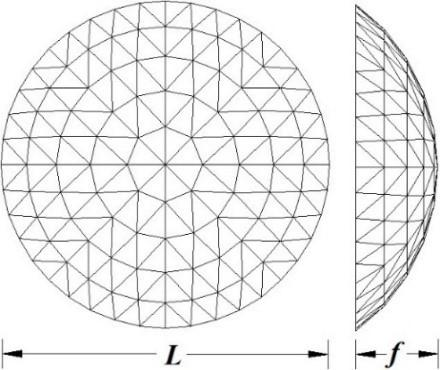Figure 1. Single-layer spherical reticulated shell

As shown in Figure 1, the spherical reticulated shell has a span L of 40m and an initial rise f of 10m, i.e. the rise-to-span ratio equals 0.25. The rods of the shell are steel tubes of three different dimensions: Ф114mm×4mm, Ф89mm×4mm and Ф60mm×3.5mm, where Ф is the outside diameter. The steel tubes are made of Q235 steel, with elastic modulus E=2.06×105N/mm2, Poisson’s ratio ν=0.26 and yield strength fy=235N/mm2.

## 2.jpg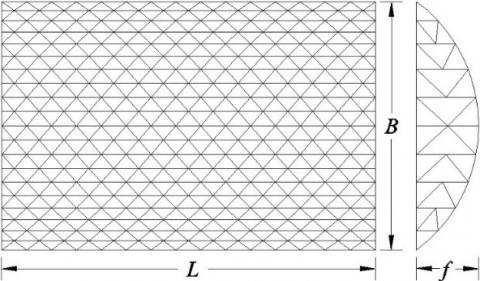Figure 2. Single-layer cylindrical reticulated shell

As shown in Figure 2, the cylindrical reticulated shell has a longitudinal length L of 45m, a span B of 30m and an initial rise f of 7.5m, i.e. the rise-to-span ratio equals 0.25. The rods of the shell are steel tubes of four different dimensions: of Ф140mm×4.5mm, Ф114mm×4mm, Ф89mm×4mm and Ф76mm×3.5mm. The other parameters of the steel tubes are the same as those of the spherical reticulated shell.

2.2 Equilibrium path

The equilibrium path of each reticulated shell can be solved nonlinearly by arc length method. It is assumed that the snow load is independent of deformation and proportionally loaded on the shell. Then, the incremental equilibrium equation can be defined as:

$K \Delta U^{(i)}=^{t+\Delta t} \lambda^{(i)} R-^{t+\Delta t} F^{i-1}$     (1)

where, $^{\prime} K$ is the tangent stiffness matrix of the shell at time $t$; $\Delta U^{(i)}$ is the iterative increment of displacement; $^{i+\Delta t} \lambda$ is the load proportional coefficient at time $t ;$ retar $F$ -is the internal. force vector of a node at time $t+\Delta t ; R$ is the \cdotload distribution vector.

Taking the sum of squares of λ and U as a variable, the arc length constraint can be generalized as:

$\alpha\left\{\left(^{t+\Delta t} \lambda^{i+1}-\Delta \lambda^{(i)}\right)+\Delta \lambda^{(i)}\right\}^{2}+U^{(i) \mathrm{T}} U^{(i)}=(\Delta l)^{2}$     (2)

where, $\Delta l$ is the iterative increment of arc length; $\alpha$ is the scale factor. If $\alpha=1,$ formula ( 2 ) is the spherical arc length method.

2.3 Grid meshing

The snow load on a reticulated shell is affected by various factors, ranging from sunlight, wind direction to heat dissipation. The distribution of snow load directly bears on the buckling path of the shell. Different distributions may cause varied degrees of decline in the bearing capacity of the shell.

As mentioned before, this paper mainly aims to disclose how the asymmetry and non-uniformity of snow load distribution affect the stability of two kinds of single-layer reticulated shells. For this purpose, the nonlinear finite-element program ANSYS was adopted to analyze the stability of the shells under different conditions, and to identify the most dangerous distribution of snow load.

Before the analysis, all the rods of each shell were meshed into BEAM188 elements, which fully demonstrate the geometry of the shell and nonlinearity of the steel. Besides, the roof load and self-weight of each shell were considered as an equivalent concentrated load acting on the nodes of the shell. Then, the equivalent concentrated load on node i can be described as:

$F_{\mathrm{i}}(x, y, z)=S_{\mathrm{k}, \mathrm{i}}(x, y, z) A_{\mathrm{i}}$     (3)

where, Ai is the equivalent area of snow load at node i; Sk,i is the standard snow pressure at node i:

$S_{\mathrm{ki}}(x, y, z)=\mu_{k, \mathrm{i}} S_{0}$     (4)

where, $\mu_{\mathrm{r}, \mathrm{i}}$ is the coefficient of snow load distribution at node i ; S0 is the basic snow pressure at the location of the shell, which mainly depends on the snow thickness.

2.4 Snow load distributions of spherical reticulated shell

(1) The snow load obeys uniform distribution (Figure 3a) or non-uniform distribution (Figure 3b) across the full span of spherical reticulated shell.

## 3.pngFigure 3. Snow load distributions across the full span

(2) The snow load obeys uniform distribution (Figure 4a) or non-uniform distribution (Figure 4b) across the half span of spherical reticulated shell.

## 4.pngFigure 4. Snow load distributions across the half span

(3) The snow load obeys uniform distribution (Figure 5a) or non-uniform distribution (Figure 5b) across the half span of the two outermost rings of spherical reticulated shell.

## 5.pngFigure 5. Snow load distributions across the half span of the two outermost rings

To identify the most dangerous distribution of snow load, it is necessary to compare the stabilities of spherical reticulated shell under the snow load distributions across the full span, the half span and the half span of the two outermost rings, respectively . Besides, the bearing capacity of the shell under each snow load distribution should be computed based on the corresponding equilibrium path. Next, the bearing capacities of the shell under uniform and non-uniform distributions of snow load should be contrasted to find the more dangerous distribution between the two. In this way, the most dangerous distribution of snow load can be identified for spherical reticulated shell.

2.5 Snow load distributions of cylindrical reticulated shell

(1) The snow load obeys uniform distribution (Figure 6a) or non-uniform distribution (Figure 6b) across the full span of cylindrical reticulated shell.

## 6.png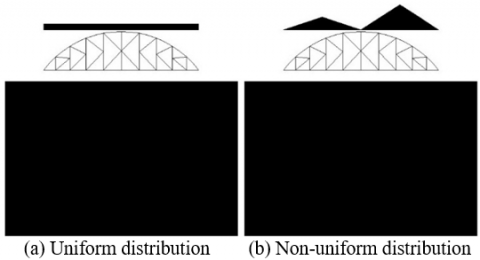Figure 6. Snow load distributions across the full span

(2) The snow load obeys uniform distribution (Figure 7a) or non-uniform distribution (Figure 7b) across the half span of cylindrical reticulated shell.

## 7.pngFigure 7. Snow load distributions across the half span

(3) The snow load obeys uniform distribution (Figure 8a) or non-uniform distribution (Figure 8b) across the half span of 2L/3 from both ends or L/3 in the middle of cylindrical reticulated shell.

## 8.pngFigure 8. Snow load distributions across the half span of 2L/3 from both ends or L/3 in the middle

In the vertical direction, the most dangerous distribution of snow load for cylindrical reticulated shell can be identified similarly as that for spherical reticulates shell.

In the horizontal direction, he most dangerous distribution of snow load for cylindrical reticulated shell can be determined in the following steps: dividing the snow load into three zones along the longitudinal length of half span; computing the bearing capacity of the shell according to the equilibrium path of each distribution; comparing the bearing capacities of the shell under different distributions.

3. Influence of Snow Load Distribution

3.1 Influence of distribution asymmetry on spherical reticulated shell

Assuming that the snow load is uniformly distributed in the vertical direction, the stabilities of spherical reticulated shell in the three regions of Figures 3a, 4a and 5a were compared in the light of bearing capacity and displacement.

The comparison results (Table 1) show that the spherical reticulated shell had the lowest bearing capacity when the snow load was distributed across the half span of the two outermost rings, which was 18.2% lower than that under half span distribution and 18.2% lower than that under full span distribution. This finding agrees with the previous results .

The main reason is that the snow load distribution affects the deformation mode and bearing capacity of the spherical reticulates shell. In terms of deformation mode, the spherical reticulated shell suffers from symmetric buckling deformation under full span distribution of snow load; the whole shell converges to the inner and lower sides, i.e. the deformation propagates along the load direction. By contrast, the spherical reticulated shell suffers from asymmetric buckling deformation under half span distribution of snow load, and the deformation is much greater on the loaded side than on the unloaded side.

In terms of bearing capacity, the two opposite sides of the spherical reticulated shell are subjected to the same load, under full span distribution of snow load; the bearing capacity of the shell is therefore relatively high. When the snow load is distributed across half span, there is no load on the opposite side of the deformation, that is, only one side is constrained. The load imbalance weakens the bearing capacity of the shell.

Through the above analysis, it is obvious that the spherical reticulated shell has a better bearing capacity under full span distribution of snow load than under half span distribution.

In addition, the spherical reticulated shell had the weakest stability, when the snow load was distributed uniformly across the half span of the two outermost rings, and had the smallest displacement at the moment of failure. That is why the most sudden buckling failure may occur in this scenario.

Table 1. Stabilities of spherical reticulated shell under different uniform distributions of snow load

 Snow load distribution Bearing capacity (kN/m2) Displacement (m) Decrement from Figure 3a (%) Figure 3a 13.11 0.207 - Figure 4a 10.73 0.183 18.2% Figure 5a 8.78 0.121 33.0%

Next, it is assumed that the snow load is non-uniformly distributed in the vertical direction. The stabilities of spherical reticulated shell in the three regions of Figures 3b, 4b and 5b were compared in the light of bearing capacity and displacement.

The comparison results (Table 2) show that the spherical reticulated shell had the lowest bearing capacity when the snow load was distributed across the half span of the two outermost rings, which was 13.3% lower than that under half span distribution and 9.6% lower than that under full span distribution. Hence, the spherical reticulated shell had the poorest stability and greatest displacement under the snow load distribution across the half span of the two outermost rings.

Table 2. Stabilities of spherical reticulated shell under different non-uniform distributions of snow load

 Snow load distribution Bearing capacity (kN/m2) Displacement (m) Decrement from Figure 3b (%) Figure 3b 9.23 0.187 - Figure 4b 9.62 0.187 -4.2% Figure 5b 8.34 0.211 9.6%

To sum up, whether the snow load distribution is uniform or non-uniform in the vertical direction, the bearing capacities of spherical reticulate shell under asymmetrical snow load distributions decreased by varied degrees from the levels under symmetrical distributions. This means the asymmetry of snow load distribution has an obvious impact on the stability of spherical reticulated shell.

3.2 Influence of distribution non-uniformity on spherical reticulated shell

The bearing capacities of spherical reticulated shell under uniform snow load distributions (Figures 3a, 4a and 5a) were compared with those under non-uniform snow load distributions (Figures 3b, 4b and 5b).

The comparison results (Table 3) show that the bearing capacities of spherical reticulated shell under non-uniform snow load distributions all decreased from than those under uniform distributions. The greatest decrement (29.6%) was observed under full span distribution of snow load (Figure 3a vs. Figure 3b), followed by that (10.3%) under half span distribution (Figure 4a vs. Figure 4b), and that (5.01%) under half span distribution of the two outermost rings (Figure 5a vs. Figure 5b). The results show that non-uniformity of snow load distribution could weaken the stability of spherical reticulated shell.

Table 3. Stabilities of spherical reticulated shell under non-uniform and uniform snow load distributions

 Snow load distribution Bearing capacity (kN/m2) Snow load distribution Bearing capacity (kN/m2) Decrement from Figure a (%) Figure 3a 13.11 Figure 3b 9.23 29.6% Figure 4a 10.73 Figure 4b 9.62 10.3% Figure 5a 8.78 Figure 5b 8.34 5.01%

To sum up, the stability of spherical reticulated shell is sensitive to the asymmetry and non-uniformity of snow load distribution. The bearing capacity and failure mode of the shell are both affected by snow load distribution.

Considering both asymmetry and non-uniformity, the most dangerous distribution of snow load for spherical reticulated shell is the non-uniform distribution across the half span of the two outermost rings. The bearing capacity of spherical reticulated shell under this distribution was 36.4% lower than that under the uniform distribution of snow load across the full span. The load-displacement curves under the two distributions are compared in Figure 9 below.

## 9.png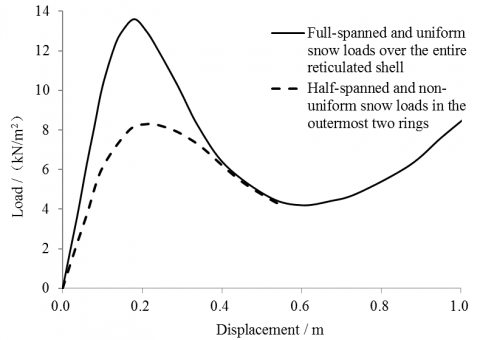Figure 9. Comparison of load-displacement curves

3.3 Influence of distribution asymmetry on cylindrical reticulated shell

Cylindrical reticulated shell is another common single-layer reticulated shell, which differs from spherical reticulated shell in load bearing features. The spatial cooperation of cylindrical reticulate shell is weaker than the spherical one.

The stabilities of cylindrical reticulated shell under uniform distributions (Figures 6a and 7a) and non-uniform distributions (Figures 6b and 7b) of snow load in the vertical direction were calculated and compared as those of spherical reticulated shell.

The comparison results (Tables 4 and 5) show that, whether the snow load obeys uniform or non-uniform distributions, the bearing capacity of cylindrical reticulated shell under half span distribution of snow load decreased from that under full span distribution; the decrement was 59.3% (Figure 6a vs. Figure 7a) when the snow load obeys uniform distribution, and 18.2% (Figure 6b vs. Figure 7b) when the snow load obeys non-uniform distribution.

Compared with that of spherical reticulates shell, the stability of cylindrical reticulated shell is severely affected by the asymmetry of snow load distribution.

Table 4. Stabilities of cylindrical reticulated shell under different uniform distributions of snow load

 Snow load distribution Bearing capacity (kN/m2) Displacement (m) Decrement from Figure 6a (%) Figure 6a 10.40 0.300 - Figure 7a 4.23 0.183 59.3%

Table 5. Stabilities of cylindrical reticulated shell under different non-uniform distributions of snow load

 Snow load distribution Bearing capacity (kN/m2) Displacement (m) Decrement from Figure 6b (%) Figure 6b 7.17 0.225 - Figure 7b 3.47 0.353 51.6%

3.4 Influence of distribution non-uniformity on cylindrical reticulated shell

The bearing capacities of cylindrical reticulated shell under uniform snow load distributions (Figures 6a and 7a) were compared with those under non-uniform snow load distributions (Figures 6b and 7b).

The comparison results (Table 6) show that the bearing capacities of cylindrical reticulated shell under non-uniform snow load distributions both decreased from than those under uniform distributions. The decrements were 31.1% and 18.0%, respectively, for Figure 6a vs. Figure 7a and Figure 6b vs. Figure 7b. Therefore, the non-uniform distribution of snow load, whether across full span or half span, can weaken the stability of cylindrical reticulated shell.

Compared with that of spherical reticulates shell, the stability of cylindrical reticulated shell is severely affected by the non-uniformity of snow load distribution.

Table 6. Stabilities of cylindrical reticulated shell under non-uniform and uniform snow load distributions

 Snow load distribution Bearing capacity (kN/m2) Snow load distribution Bearing capacity (kN/m2) Decrement from Figure a (%) Figure 6a 10.40 Figure 6b 7.17 31.1% Figure 7a 4.23 Figure 7b 3.47 18.0%

3.5 Influence of distribution areas on cylindrical reticulated shell

The above analysis shows that the most dangerous snow load distribution for cylindrical reticulated shell is the non-uniform distribution across the half span. To further explore the dangerous distribution of snow load, the snow load was divided into three areas in the longitudinal direction of the half span: 2L/3 from the left end, 2L/3 from the right end, and L/3 in the middle of cylindrical reticulated shell (Figures 8a and 8b). The bearing capacities of the cylindrical reticulated shell were calculated for each of the three areas of snow load.

The calculation results (Table 7) show that the bearing capacity of the cylindrical reticulated shell in the middle was 19.8% smaller than that at the two ends, when the snow load obeys non-uniform distribution across the half span. This is because the rods in middle area are less confined than those at the two ends.

Table 7. Stabilities of cylindrical reticulated shell in different areas under non-uniform distribution of snow load across the half span

 Snow load distribution Bearing capacity (kN/m2) Snow load distribution Bearing capacity (kN/m2) Decrement from Figure a (%) Figure 8a 3.404 Figure 8b 2.729 19.8%

To sum up, the stability of cylindrical reticulated shell is more sensitive to the asymmetry and non-uniformity of snow load distribution than that of spherical reticulated shell. Considering both asymmetry and non-uniformity, the most dangerous distribution of snow load for cylindrical reticulated shell is the non-uniform distribution across the half span in the middle. The bearing capacity of spherical reticulated shell under this distribution was 73.8% lower than that under the uniform distribution of snow load across the full span. The load-displacement curves under the two distributions are compared in Figure 10 below.

## 10.png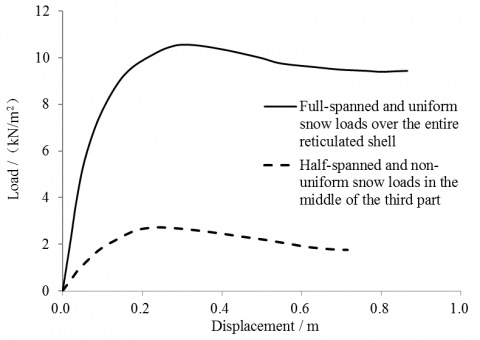Figure 10. Comparison of load-displacement curves

4. Conclusions

The stability of spherical reticulated shell under asymmetrical snow load distributions decreased by varied degrees from the levels under symmetrical distributions. The asymmetry of snow load distribution has an obvious impact on the stability of spherical reticulated shell and an even greater impact on that of cylindrical reticulated shell.

In the vertical direction, the non-uniformity of snow load distribution greatly reduces the bearing capacity of spherical reticulated shell. The reduction effect cannot be neglected. Moreover, the non-uniformity of snow load distribution exerts an even greater reduction effect on the stability of cylindrical reticulated shell.

Considering both asymmetry and non-uniformity, the most dangerous distribution of snow load for spherical reticulated shell is the non-uniform distribution across the half span of the two outermost rings. The bearing capacity of spherical reticulated shell under this distribution was 36.4% lower than that under the uniform distribution of snow load across the full span.

Similarly, the most dangerous distribution of snow load for cylindrical reticulated shell is the non-uniform distribution across the half span in the middle. The bearing capacity of spherical reticulated shell under this distribution was 73.8% lower than that under the uniform distribution of snow load across the full span. Without considering the asymmetry and non-uniformity of snow load distribution, the stabilities of spherical reticulated shell and cylindrical reticulated shell will be overestimated, which may lead to structural insecurity.

Acknowledgements

This work was supported by Natural Fund of Hebei Province (no. E2018204019), Education Youth Fund of Hebei Provincial Education Department (no. QN2018256) and Science and Engineering Fund of Hebei Agricultural University (no. LG201713 and ZD201704).

References

 Gioncu, V., Balut, N. (1992). Instability behaviour of single layer reticulated shells. International Journal of Space Structures, 7(4): 243-252. https://doi.org/10.1177/026635119200700402

 Liu, H., Zhang, W., Yuan, H. (2016). Structural stability analysis of single-layer reticulated shells with stochastic imperfections. Engineering Structures, 124: 473-479. https://doi.org/10.1016/j.engstruct.2016.06.046

 He, Y., Zhou, X., Liu, D. (2014). Research on stability of single-layer inverted catenary cylindrical reticulated shells. Thin-Walled Structures, 82: 233-244. https://dx.doi.org/10.1016/j.tws.2014.04.017

 Fan, F., Cao, Z., Shen, S. (2010). Elasto-plastic stability of single-layer reticulated shells. Thin-Walled Structures, 48(10-11): 827-836. https://doi.org/10.1016/j.tws.2010.04.004

 Takiuchi, Y., Kato, S., Nakazawa, S. (2017). Buckling strength maximization of single layer free-form reticulated shells. Journal of Structural and Construction Engineering, 733(82): 441-450. https://doi.org/10.3130/aijs.82.441

 Taniguchi, Y., Matsui, T., Yoshinaka, S. (2018). Comparison study of elasto-plastic behavior on static and dynamic responses for single layer lattice domes under vertical loading. Journal of Structural and Construction Engineering, 747(83): 709-716. https://doi.org/10.3130/aijs.83.709

 Jung, H.M., Yoon, S.H., Lee, D.W. (2016). Reinforcement effects of buckling member for single-layer latticed dome. Journal of the Korean Association for Spatial Structures, 16(4): 45-52. https://doi.org/10.9712/KASS.2016.16.4.045

 López, A., Puente, I., Serna, M.A. (2007). Direct evaluation of the buckling loads of semi-rigidly jointed single-layer latticed domes under symmetric loading. Engineering structures, 29(1): 101-109. https://doi.org/10.1016/j.engstruct.2006.04.008

 Ma, H., Wang, W., Zhang, Z., Fan, F. (2017). Research on the static and hysteretic behavior of a new semi-rigid joint (BCP Joint) for single-layer reticulated structures. Journal of the International Association for Shell and Spatial Structures, 58(2): 159-172. https://doi.org/10.20898/j.iass.2017.192.817

 Koo, C.M., Jung, H.M., Kim, C.H. (2012). A study on the stability of the single-layer latticed dome during erection using the step-up method. Journal of the Korean Association for Spatial Structures, 12(4): 109-118. https://doi.org/10.9712/KASS.2012.12.4.109

 Geis, J., Strobel, K., Liel, A. (2012). Snow-induced building failures. Journal of Performance of Constructed Facilities, 26(4): 377-388. https://doi.org/10.1061/(ASCE)CF.1943-5509.0000222

 Liel, A.B., DeBock, D.J., Harris, J.R., Ellingwood, B.R., Torrents, J.M. (2017). Reliability-based design snow loads. II: Reliability assessment and mapping procedures. Journal of Structural Engineering, 143(7): 04017047. https://doi.org/10.1061/(ASCE)ST.1943-541X.0001732

 Kozak, D.L., Liel, A.B. (2015). Reliability of steel roof structures under snow loads. Structural Safety, 54: 46-56. https://doi.org/10.1016/j.strusafe.2015.02.004

 Meløysund, V., Lisø, K.R., Siem, J., Apeland, K. (2006). Increased snow loads and wind actions on existing buildings: reliability of the Norwegian building stock. Journal of Structural Engineering, 132(11): 1813-1820. https://doi.org/10.1061/(ASCE)0733-9445(2006)132:11(1813)

 Soltis, L.A. (1984). Low-rise timber buildings subjected to seismic, wind, and snow loads. Journal of Structural Engineering, 110(4): 744-753. https://doi.org/10.1061/(ASCE)0733-9445(1984)110:4(744)

 Takahashi, T., & Ellingwood, B. R. (2005). Reliability-based assessment of roofs in Japan subjected to extreme snows: Incorporation of site-specific data. Engineering Structures, 27(1): 89-95. https://doi.org/10.1016/j.engstruct.2004.09.001

 Martin, R., Delatte, N.J. (2001). Another look at Hartford civic center coliseum collapse. Journal of Performance of Constructed Facilities, 15(1): 31-36. https://doi.org/10.1061/(ASCE)0887-3828(2001)15:1(31)

 Smith, E.A., Epstein, H.I. (1980). Hartford Coliseum roof collapse: Structural collapse sequence and lessons learned. Civil Engineering-ASCE, 50(4): 59-62.

 Kato, S., Iwamoto, T. (2017). Buckling and reliability analysis of single layer grid dome with diagonal brace under snow load. Journal of the International Association for Shell and Spatial Structures, 58(3): 207-225. http://doi.org/10.20898/j.iass.2017.193.862

 GB50009-2012. (2012). Load Code for the Design of building Structures. Beijing: China Architecture and Building Press.

 Li, Y., Yuan, X.F. (2014). Numerical simulation of snow drifting and research on snow uneven distribution coefficient of long-span spherical shell roofs. Journal of Building Structures, 35(10): 130-136.

 Thiis, T.K., Gjessing, Y. (1999). Large-scale measurements of snowdrifts around flat-roofed and single-pitch-roofed buildings. Cold Regions Science and Technology, 30(1-3): 175-181. https://doi.org/10.1016/S0165-232X(99)00021-X

 Du, W.F., Gao, B.Q., Dong, S.L. (2014). Research on the most dangerous snow distribution on single-layer spherical latticed shells. Engineering Mechanics, 31(3): 83-86, 92. https://doi.org/10.6052/j.issn.1000-4750.2012.10.0751

 Zhang, C.L., Li, Z.L., Zhao, Y., Shao, F.J. (2004). Effect of unsymmetrical loading distribution to the stability of single-layer latticed domes. Journal of Chongqing Jianzhu University, 26(2): 63-67. https://doi.org/10.11835/j.issn.1674-4764.2004.02.015

 Standard, C. (2010). Technical Specification for Space Frame Structures. JGJ7-2010. Beijing: Chinese Standard.